[Next]: Approximation and optimization of curved mechanical
 [Up]: Project descriptions
 [Previous]: Quasilinear parabolic systems with discontinuous coefficients
[Contents]   [Index]

## Numerical simulation and optimization of SiC single crystal growth by sublimation from the gas phase

Collaborator: O. Klein , P. Philip , J. Schefter , J. Sprekels

Cooperation with: Ch. Meyer, A. Rösch, F. Tröltzsch (Technische Universität Berlin) , K. Böttcher, D. Schulz, D. Siche (Institut für Kristallzüchtung (IKZ), Berlin)

Supported by: BMBF: Numerische Simulation und Optimierung der Züchtung von SiC-Einkristallen durch Sublimation aus der Gasphase'' (Numerical simulation and optimization of SiC single crystal growth by sublimation from the gas phase);

DFG-Forschungszentrum Mathematik für Schlüsseltechnologien'' (Research Center Mathematics for Key Technologies'')

Description:

Owing to numerous technical applications in electronic and optoelectronic devices, such as lasers, diodes, and sensors, the industrial demand for high quality silicon carbide (SiC) bulk single crystals remains large. It is still a challenging problem to grow sufficiently large SiC crystals with a low defect rate. Sublimation growth of SiC bulk single crystals via physical vapor transport (PVT), also known as the modified Lely method, has been one of the most successful and most widely used growth techniques of recent years. It is the goal of this project to use mathematical modeling and numerical simulation to help optimizing the PVT method.

During PVT, a graphite crucible (see Figure 1) is placed in a low-pressure inert gas atmosphere consisting of argon. The crucible is then intensely heated, e.g., by induction heating, to temperatures up to 3000 K. Inside the crucible, polycrystalline SiC source powder sublimates, adding molecules made up of silicon and carbon to the gas phase. For the moment, we abuse notation to denote the total of the added species as the SiC gas, notwithstanding the fact that it consists predominantly of other molecules than SiC. The SiC gas diffuses through the cavity from the powder to the SiC seed. As the single crystalline seed is kept at a temperature below that of the SiC source, SiC gas crystallizes on the seed, which thereby grows into the reactor.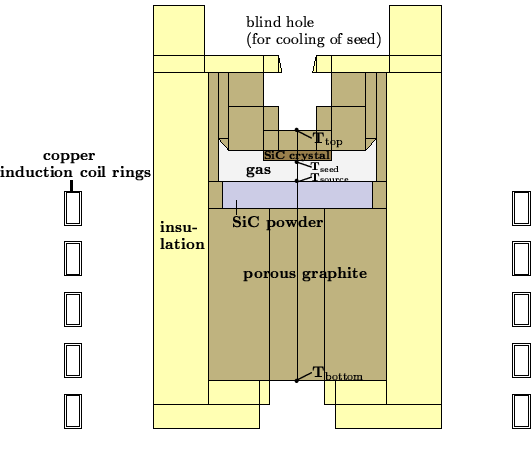The physical and mathematical modeling of the growth process leads to a highly nonlinear system of coupled partial differential equations. In addition to the kinetics of a rare gas mixture at high temperatures, one has to consider heat transport by conduction and radiation, reactive matter transport through porous and granular media, and different kinds of chemical reactions and phase transitions. Moreover, several free boundaries occur in the model, e.g., due to the growing crystal and due to the sublimating source powder. The main control parameters with respect to an optimization of the crystal growth process are the design of the growth apparatus, the position of the induction coil, the heating power, and the inert gas pressure.

Within the research year 2002, the conditions for the growth of the crystal have been further investigated. As a first approximation, one can assume that the pressure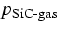of the SiC gas at the surface of the crystal is identical to the corresponding equilibrium pressure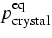. At the powder surface, one would then make the corresponding assumption. However, this approach supposes that the growth process is limited by the transport through the gas phase and it omits the influence of the growth kinetics.

To take into account the influence of the growth kinetics, one can use a Hertz-Knudsen formula to determine the mass flux from the gas to the crystal: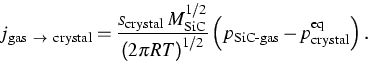(1)
Here,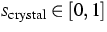is a sticking coefficient. It denotes the probability for a molecule that collides with the surface to stick to that surface and be absorbed. Moreover,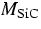is the molar mass of SiC (in kg/kmol), and R is the universal gas constant. On the powder surface, one would use a similar condition with some effective sticking coefficient, taking into account that the surface is jagged and porous.

If the model is to include the observed graphitization of the powder source, it becomes necessary to consider the different constituents of the SiC gas'', consisting of Si,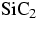,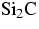,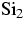, SiC, etc. Instead of simple sublimation, one has to consider several chemical reactions occurring at the surfaces. Then, instead of equilibrium pressures for the different components, one merely has relations between the different partial pressures in the equilibrium, resulting from the mass action laws of the considered chemical reactions. For example, from the reactions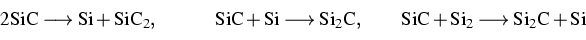involving solid SiC and some gas species, the mass action laws yield that there are temperature-dependent functions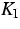,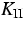, and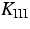such that for the partial pressures of a gas mixture in equilibrium with the SiC crystal, it holds that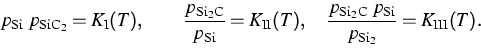Further research is ongoing to formulate the corresponding mass action laws for reactions changing the composition of the surface, to model the kinetics of the chemical reactions, and to determine the involved material parameters.

The heat sources caused by induction heating are computed via an axisymmetric complex-valued magnetic scalar potential that is determined as the solution of an elliptic PDE using the imposed voltage as input data. The scalar potential enables one to calculate the resulting current density and thus the heat sources [2, 3, 4].

The transient nonlinear and nonlocal heat transport equations are discretized using an implicit Euler scheme in time and a finite volume method in space. Within the period covered by this report, existence and uniqueness results for the solution to the resulting nonlinear and nonlocal discrete scheme have been established. Moreover, discrete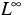-L1 a priori estimates have been proved .

Within the covered research period, the simulation software WIAS-HiTNIHS (see page) has been installed at the IKZ. Figure 2 depicts results of a numerical simulation of the heating process for the configuration of Figure 1 which we published in . The angular frequency used for the induction heating is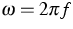, where f=10 kHz. The average total power P is prescribed according to the following linear ramp: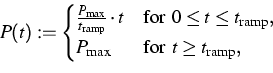(2)
where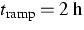. Each simulation starts at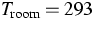K.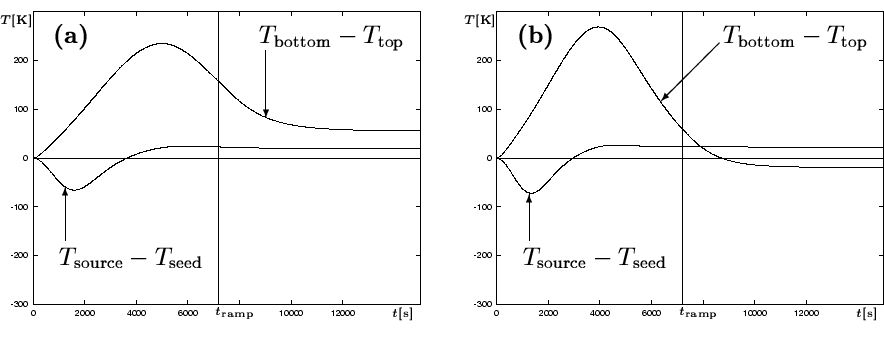We investigate the relation between the temperature differences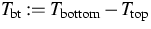and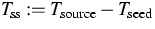. The relation between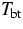and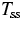is of importance, as in physical growth experiments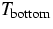and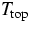are measured, andis often used as an indicator forwhich is not accessible to direct measurements, but crucial for the growth process. Figure 2 shows that in both cases,is no indicator forbeforehas reached its quasi-stationary final state, but, more importantly, even in the stationary state at growth temperature,is almost three times as large asfor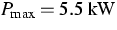, andandhave different signs for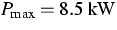.The numerical experiments show that for the considered configuration, there is generally no easy relation betweenand.Even though there might be configurations where the situation is better, for each real growth system, the validity of usingas an indicator forneeds to be verified by some other method (e.g., numerical simulation).

References:

1. M. PONS, M. ANIKIN, K. CHOUROU, J.M. DEDULLE, R. MADAR, E. BLANQUET, A. PISCH, C. BERNARD, P. GROSSE, C. FAURE, G. BASSET, Y. GRANGE, State of the art in the modelling of SiC sublimation growth,
Materials Science and Engineering B, 61-62 (1999), pp. 18-28.
2. O. KLEIN, P. PHILIP, Correct voltage distribution for axisymmetric sinusoidal modeling of induction heating with prescribed current, voltage, or power,
IEEE Transactions on Magnetics, 38 (2002), pp. 1519-1523.
3., Transient numerical investigation of induction heating during sublimation growth of silicon carbide single crystals,
J. Crystal Growth, 247 (2002), pp. 219-235.
4., Induction heating during SiC growth by PVT: Aspects of axisymmetric sinusoidal modeling,
in: Proceedings of the 10-th International IGTE Symposium on Numerical Field Calculation in Electrical Engineering, Graz, Austria, September 16-18, 2002, CD-ROM, ISBN: 3-901351-65-5, pp. 271-276.
5., Transient temperature phenomena during sublimation growth of silicon carbide single crystals, WIAS Preprint no. 755, 2002, to appear in: J. Crystal Growth.
6. P. PHILIP, Transient numerical simulation of sublimation growth of SiC bulk single crystals. Modeling, finite volume method, results, PhD thesis, submitted to the Institut für Mathematik, Humboldt-Universität zu Berlin, 2002.

 [Next]: Approximation and optimization of curved mechanical
 [Up]: Project descriptions
 [Previous]: Quasilinear parabolic systems with discontinuous coefficients
[Contents]   [Index]

LaTeX typesetting by I. Bremer
5/16/2003